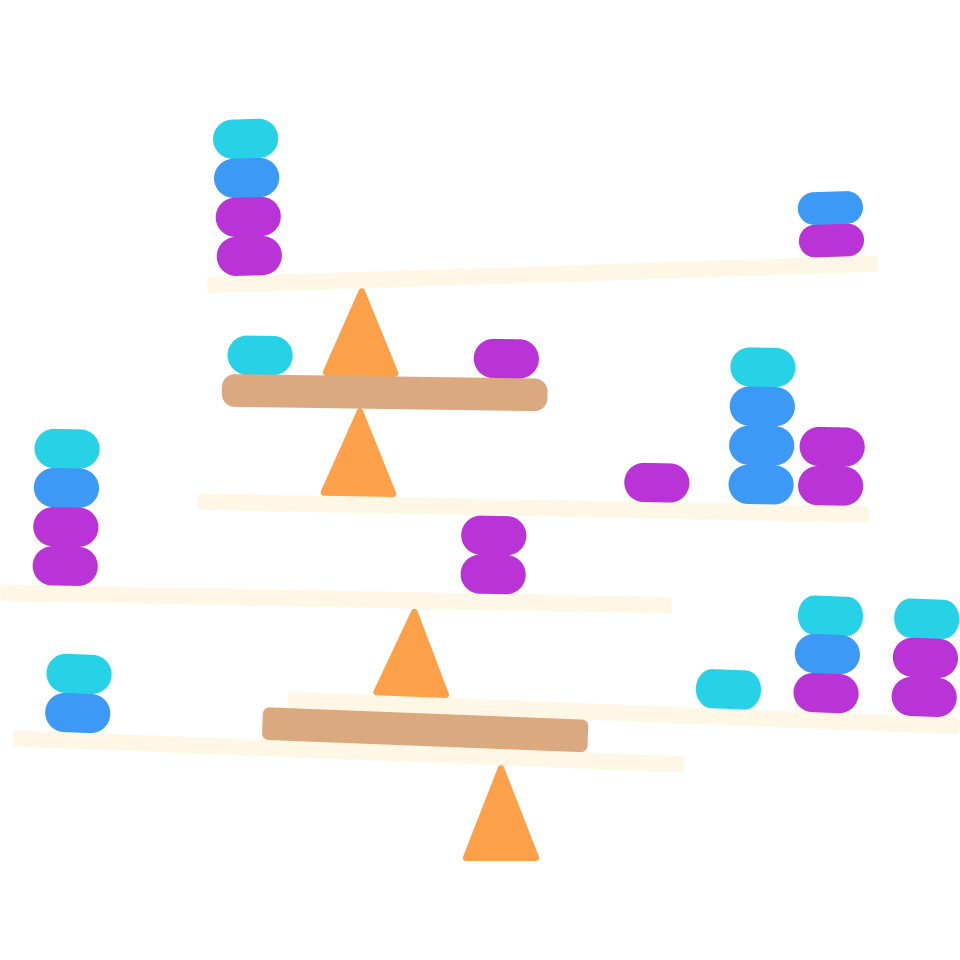# Magic Sum Puzzles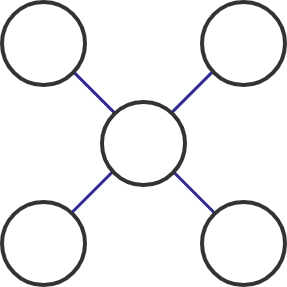Place the numbers 1 to 5 in the circles above, one number per circle, so that the sum of numbers on each of the two diagonal lines is 9.

What number goes in the middle?

# Magic Sum Puzzles

Puzzles like the previous one can be solved by just playing around with the numbers and figuring out where they fit.

However, rather than just doing arithmetic, we can think about the process involved in doing arithmetic—that is, do algebra—to make puzzles easier to solve. This also allows us to see the mechanics behind how they work.

In the next few questions, we'll examine various aspects of the problem below to see how algebraic thinking helps us solve problems like this.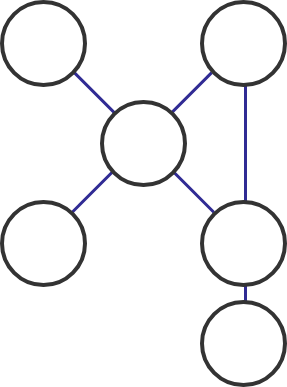Place all the numbers from 1 to 6 (one in each circle) so that the sum of numbers on each of the three marked lines comes out to be 12.

# Magic Sum Puzzles

We'll spend the next few questions considering the puzzle below:Place all the numbers from 1 to 6 (one in each circle) so that the sum of numbers on each of the three blue lines comes out to be 12.

It can help to break the puzzle up into regions and compare them; one method is shown below. When we calculate all the sums described in the puzzle, what is the most important difference between the circles marked in red and those marked in green?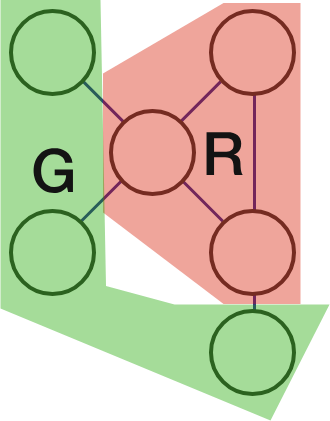# Magic Sum PuzzlesPlace all the numbers from 1 to 6 (one in each circle) so that the sum of numbers on each of the three marked lines comes out to be 12.

In order to analyze the puzzle, it helps to split the circles into an "inner section" where the sums overlap (marked red below) and an "outer section" (marked green below).If $R$ is the sum of numbers in the red section and $G$ is the sum of numbers in the green section, which equation is true? (Remember we're using the numbers 1 through 6.)

# Magic Sum Puzzles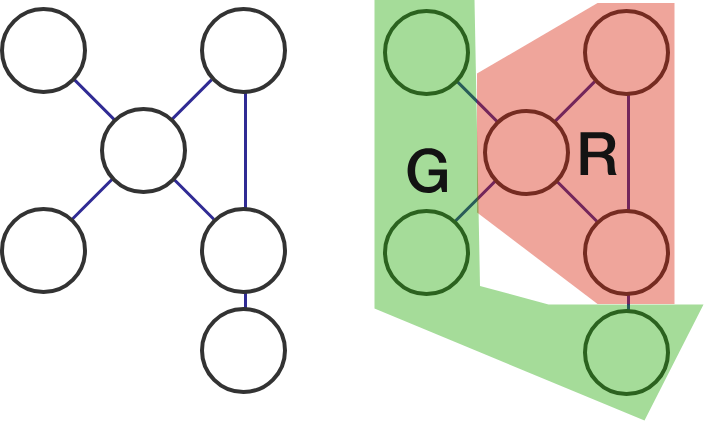Place all the numbers from 1 to 6 (one in each circle) so that the sum of numbers on each of the three marked lines comes out to be 12.

When analyzing a puzzle with algebra, it helps to think of the same problem from multiple perspectives. Instead of thinking of all the numbers together, let's think of all the sums together. There are three sums, each 12, so the total of the sums is $12 \times 3 = 36 .$

Which equation must also be true?

# Magic Sum PuzzlesBased on the previous problems, we now know that $R + G = 21$ and $2R + G = 36 .$ We can use algebra to combine these statements and figure out what $R$ and $G$ are.

What is $R?$ (Hint: Subtract the first equation from the second.)

# Magic Sum PuzzlesPlace all the numbers from 1 to 6 (one in each circle of the original puzzle) so that the sum of numbers on each of the three marked lines comes out to be 12.

We have now learned that $R$ sums to 15. What number must go in the top left circle (in green) if 4 is in the top right?

# Magic Sum Puzzles

The algebra has led us to knowing where the sets $1, 2, 3$ and $4, 5, 6$ are.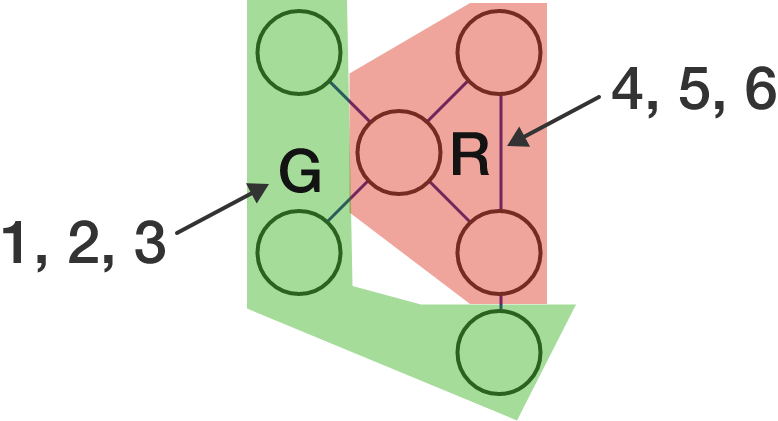Past this point, there are multiple options. With the last question we tried placing the 4 in the upper right corner, but it can go anywhere in the red region; this leads to six different answers.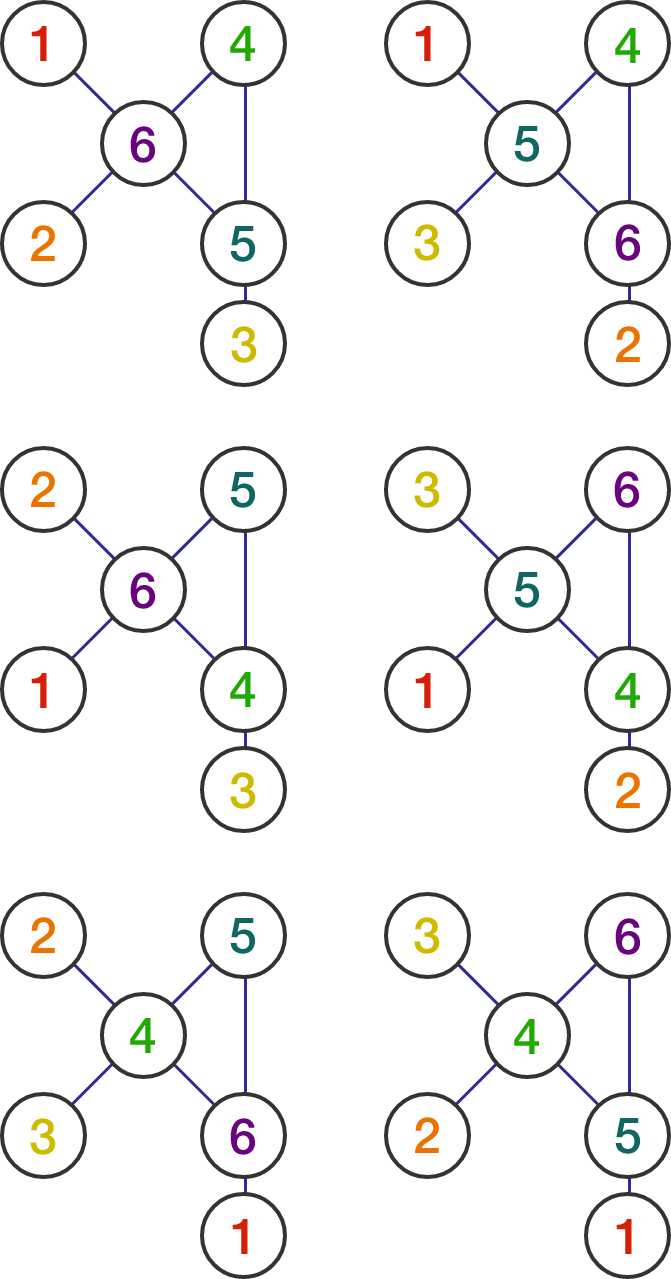While it'd be possible to find all the solutions above by guessing, algebra narrows down our search and makes it more systematic; it helps us know we have all the solutions to the puzzle with greater confidence.

# Magic Sum Puzzles

Let's change up the goal slightly and practice thinking algebraically!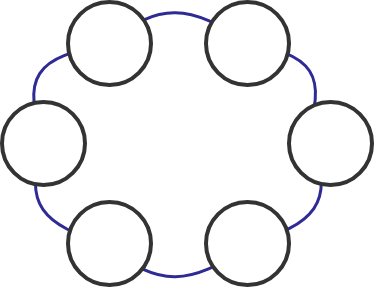Suppose you want to fill the puzzle above with the numbers 1 through 6 (one each circle) so that every set of three circles next to each other add up to the same sum.

Is this possible to do?

×

Problem Loading...

Note Loading...

Set Loading...